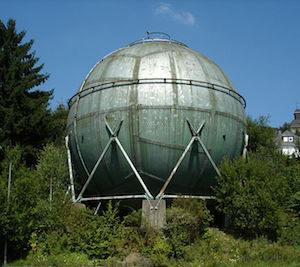# The field distorting sphereAn earthed metallic sphere is kept in a uniform electric field $E_0 \hat{k}$. If $V(r, \theta ,\phi )$ denotes the potential function for the region outside the sphere then the value of $\displaystyle \frac{V \left( 2R, \frac{\pi }{3} , \frac{ \pi }{2} \right) }{ V \left( 3R, \frac{-\pi }{3} , \frac{ 2\pi }{3} \right) }$ can be expressed as $\dfrac{a}{b}$ for some positive coprime integers $a,b$.

Evaluate $a+b$.

Details and Assumptions:

• $\hat{k}$ is the unit vector along the direction of $z$ axis in a normal right handed Cartesian coordinate system.

• Reference potential i.e. $(V=0)$ is obviously the sphere since it is grounded.

• $R$ is the radius of the sphere.

###### Image credit: Wikipedia Bob lonescu
×

Problem Loading...

Note Loading...

Set Loading...